# Lesson 2 In-Class Discussion ✅

(danny iskandar) #717

Free GPU during this course?

Hi everybody, I heard Jeremy mentioned there is free GPU ? Crestle? that are free using VC moneys at our disposal. Which one? How can we use it? Thank you.
Right now I am just using google colab, but nice to have an option.

#718

all the info you need is here witth this link top post:

(Alvaro) #719

@bluesky314, If you’re using crestle the sudo installs for the animation are not needed, just replace html5 with jshtml like this:

``````from matplotlib import animation, rc
rc('animation', html='jshtml')``````

(Ramesh Kumar Singh) #720

Hi everyone, I came across few terms and wanted to know bit more about them. One of them is dropouts.
It would be really great if some one can help me understand what is dropout and how does it penalise training loss and helps prevent over-fitting.

(Sergey) #721

You can watch lesson 4 from the MOOC. here is the link to wiki of that lesson. Dropout is described after minute 5. And further questions about dropout should be posted in advanced category since they were not covered during Lesson 2

Hi all, question re: normalization of data:

The mnist example from class doesnt do any normalization for the resnet18 model…is there a reason for that? And if you were to, should you use data.normalize(mnist_stats) instead of data.normalize(imagenet_stats)?

My understanding is that you should normalize the new data by the stats of the data used to train the pretrained model used for transfer learning…but how do you know what data was used for training the pretrained models (ex. resnet18, resnet34, resnet50, etc.)?

Thanks!

(Ravi S. Rāmphal) #724

I was basically asking about multiclassification and segmentation, which is discussed in Lesson 3.

(Rick N) #725

Thanks for the ideas; I was only tweaking lr and # of epochs; I hadn’t yet gotten into ps and wd. I tried some ps changes today but still no luck, so I’m going to wait until I understand it better! Here’s a post I replied to:

(Francisco Ingham) #726

What about weight decay? Have you tried increasing it?

(Rick N) #727

Damn, Francisco, you keep making me push the envelope!- which I appreciate… no, I didn’t even know where to set wd but now I’m trying it. But since I’m just shooting in the dark can you suggest how much to increase it? I just ran a quick test with wd=.01 (which I thought was the default) and it seems to have decreased my train_loss, which I don’t understand.

(Francisco Ingham) #728

That is the default actually. How much did the training loss decrease? Probably this is due to randomness in training and not specially meaningful. What if you try 2e-2, 3e-2, 4e-2? That should increase your training loss and allow you to train more epochs with a decreasing validation loss (maybe achieving a lower validation loss than before at the end of your training).

(Rick N) #729

I ran earlier with:
learn = create_cnn(data, models.resnet34, ps=0.1, metrics=error_rate)
learn.fit_one_cycle(24, max_lr=slice(1e-4,1e-2))
and got for the 8th epoch:
8 0.193530 0.075071 0.013333 (00:07)

Then I ran:
learn = create_cnn(data, models.resnet34, ps=0.1, wd=.01, metrics=error_rate)
learn.fit_one_cycle(8, max_lr=slice(1e-4,1e-2))
and got:
8 0.094480 0.009196 0.000000

So both losses were way down.

Then because you said to increase weight decay I tried:
learn = create_cnn(data, models.resnet34, ps=0.1, wd=.1, metrics=error_rate)
and got: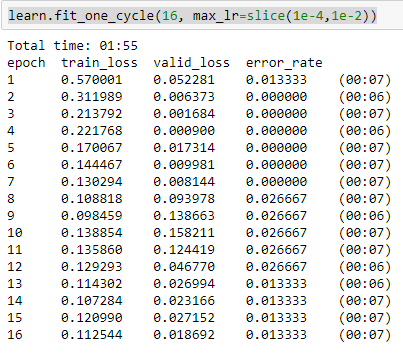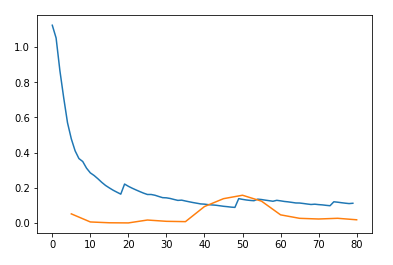I really don’t understand what’s going on…

I should note: this is generally what I’ve seen with many variations in lr and epochs - the training loss is almost always higher than validation loss, and often much higher. Error rate is usually quite good, so it seems like the model is decent, but consistently underfitting.

(Rick N) #730

Ah, this is interesting - following your suggested wd values:
learn = create_cnn(data, models.resnet34, ps=0.1, wd=0.04, metrics=error_rate)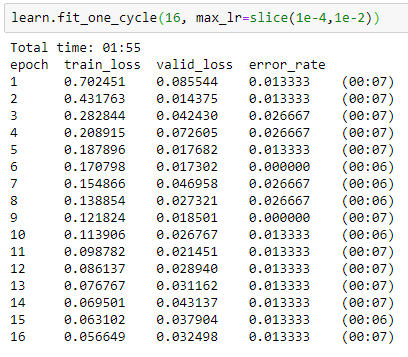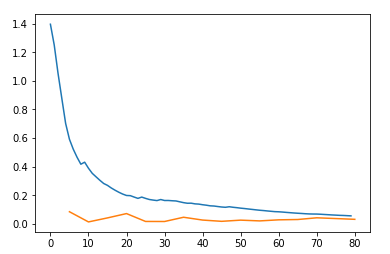(Francisco Ingham) #731

Wait I’m sorry I reread your original question and realized I read it wrong when I first answered it. You want to get your training loss lower than your validation loss so you should decrease dropout and/or weight decay since both of them make it harder for your training loss to decrease. Could you try wd=0.001? That should help!

(Rick N) #732

The funny thing is, I just ran:
learn = create_cnn(data, models.resnet34, ps=0.1, wd=0.04, metrics=error_rate)
for 24 epochs this time, and finally got train_loss < valid_loss!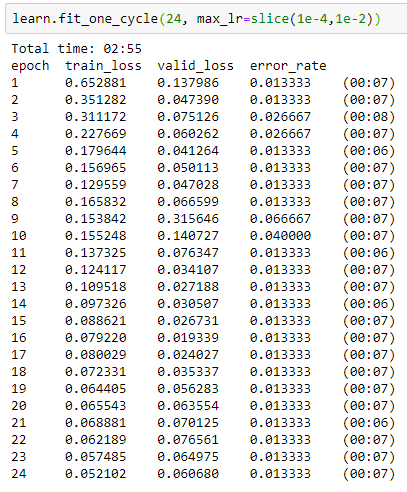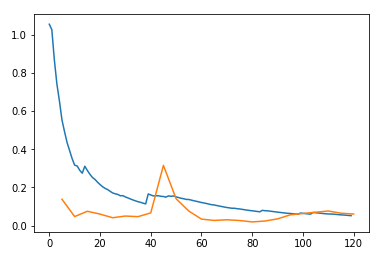So that feels like success, but I don’t understand it! Does it make sense to you?

I’ll run with wd=0.001 now.

(Francisco Ingham) #733

You are using 1/5 the default probability of dropout (0.5) that’s why. Now try with ps=0.1 and wd=0.001 and we should see some nice overfitting.

(Rick N) #734

learn = create_cnn(data, models.resnet34, ps=0.1, wd=0.001, metrics=error_rate)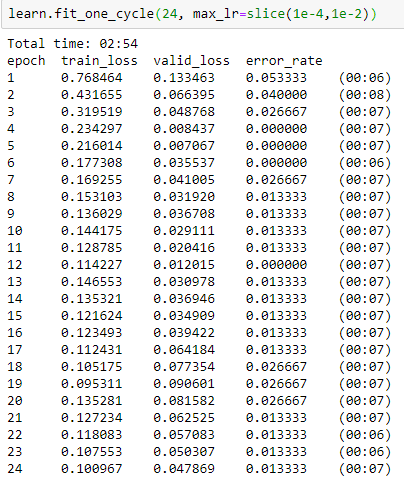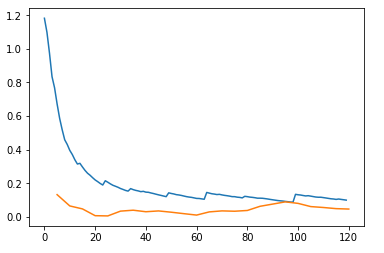…so wd=0.04 looks like the optimum.

(Francisco Ingham) #735

Weird. Can you try with wd=0?

(Rick N) #736

Here you go:

learn = create_cnn(data, models.resnet34, ps=0.1, wd=0.0, metrics=error_rate)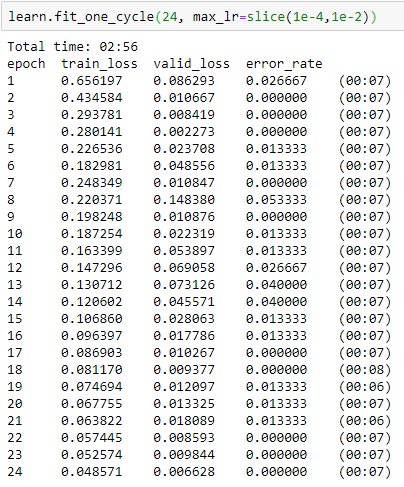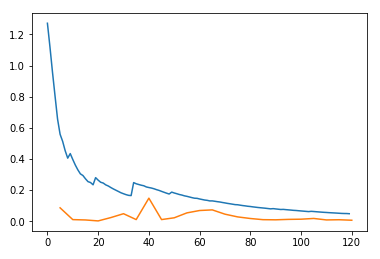Not as good as wd=0.04

(Francisco Ingham) #737

We should continue talking about this in another thread. Could you create one and tag me please?